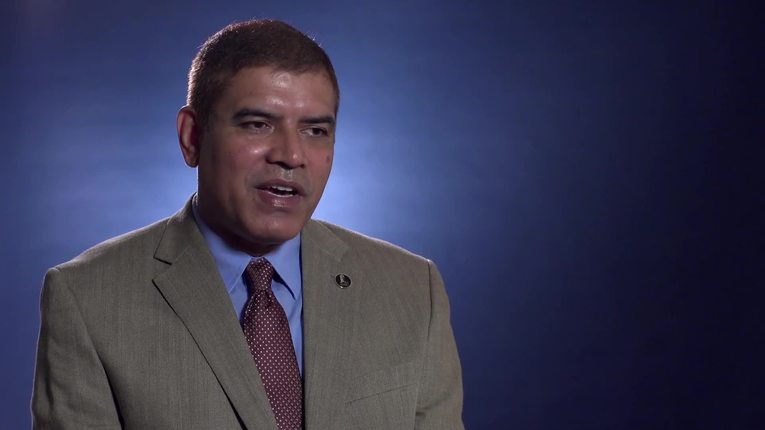# Mathematicians

OverviewJoin Vault Gold to watch these Q&A videos

A mathematician solves or directs the solution of problems in higher mathematics, including algebra, geometry, number theory, logic, and topology. Theoretical mathematicians work with the relationships among mathematical forms and the underlying principles that can be applied to problems, including electronic data processing and military planning. Applied mathematicians develop the techniques and approaches to problem solving in the physical, biological, and social sciences. Approximately 2,580 mathematicians are employed in academic and nonacademic settings in the United States. In addition, 51,250 mathematical science teachers are employed by colleges and universities across the country.

Salary Range

\$50,000 to \$100,000+

Minimum Education Level

Doctorate

Required

Outlook

Much Faster than the Average
Personality Traits

Conventional

Realistic

Scientific